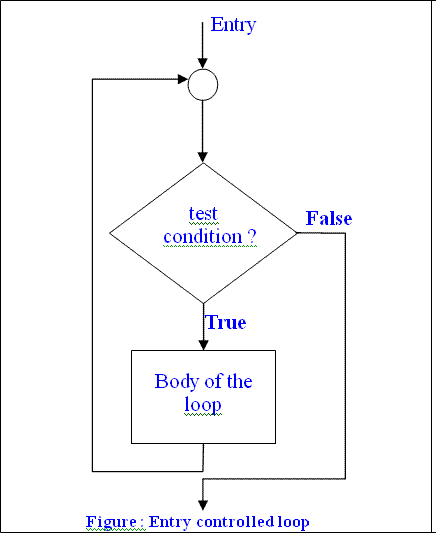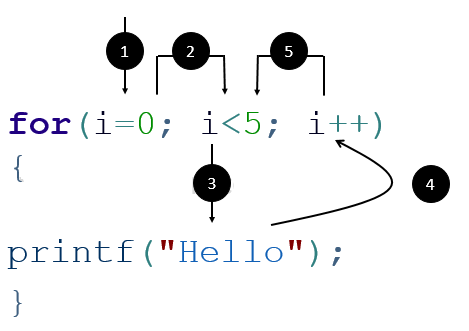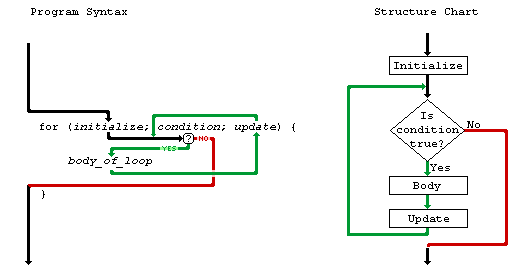• An entry control loop checks the condition at the time of entry and if condition or expression becomes true then control transfers into the body of the loop.
• Such type of loop controls entry to the loop that’s why it is called entry control loop.• The following are the main looping statements available in this category:

### While loop

• The while loop is the simplest looping structure
• It is of entry control looping structure
• If we have to execute some statements repeatedly as long as certain condition become true we can use the while loop.
• In the while loop the given expression will be check at the time of the loop entry, if given expression becomes true then control will transfer into the loop body.
• The statement inside the loop body will be executed.
• The counter value will be modified and again given expression will be checked to enter in to the loop.
• This process will continue until the given expression become false.
• The while loop contains only expression part ,initialization will be done before the while loop

Syntax:

``````while(condition)
{
//loop body
}``````

Example:

``````while( i <= 10)
{
printf("\n  Value of I is %d", i) ;
i++ ;      // modify counter value
}``````
• The following program demonstrates use of while loop to print multiplication table.
``````/* program to print multiplication table using while loop. */

#include "stdio.h"
#include "conio.h"

void main()
{
int n, i, ans;
clrscr();

printf("\n Enter No. to print Table : ");
scanf("%d", &n);

i = 1 ;     // set counter value to 1

while( i <= 10)
{
ans = n * i;
printf("\n  %d * %d = %d", n,i, ans) ;

i++ ;      // modify counter value
}
getch();
}``````

### For loop

• For loop is the most powerful and flexible looping structure.
• We can perform any complex task using for loop.
• It has the following from:

Syntax:

`````` for ( initialization ; condition ; inc/dec)
{
// loop body
}``````

Example:• For loop contains three different parts: initialization, condition and inc/dec part.
• When the loop executes for the first time, initialization of the counter variable will be done after that expression given into the condition part is tested, if test expression evaluate to true, control will enter into the loop body.
• The statements inside the loop will be executed.• After that control will transfer to the third part where counter value will be either incremented or decremented.
• With the modified counter value test expression will be check again to enter into the loop.
• This process will be repeated until condition becomes false.
• Once the condition becomes false, the loop will be skipped and statement following by for loop will be executed.
• The initialization will be performed only once when loop encounters first time.
• For loop also allows specify multiple condition as well as complex expression to be tested.
• We can also skip any of the part of the loop as per our requirement.
• The following example provides multiple initialization and expression to be tested.
• for loop can also be executed either in straight forward or in reverse manner similar to while loop.
• The following is the same program to print multiplication table using for loop.
``````/* program to print multiplication table using for loop. */

#include "stdio.h"
#include "conio.h"

void main()
{
int n, i, ans;
clrscr();

printf("\n Enter No. to print Table : ");
scanf("%d", &n);

for( i = 1; i <=10 ; i++ )
{
ans = n * i;
printf("\n  %d * %d = %d", n,i, ans) ;
}
getch();
}``````